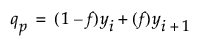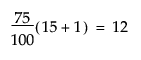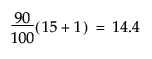Publication date: 11/10/2021

## Statistical Details for Quantiles

This section describes how quantiles are computed.

To compute the pth quantile of n nonmissing values in a column, arrange the n values in ascending order and call these column values y1, y2, ..., yn. Compute the rank number for the pth quantile as p / 100(n + 1).

If the result is an integer, the pth quantile is that rank’s corresponding value.

If the result is not an integer, the pth quantile is found by interpolation. The pth quantile, denoted qp, is defined as follows:where:

n is the number of nonmissing values for a variable

y1, y2,..., yn represents the ordered values of the variable

yn+1 is taken to be yn

i is the integer part and f is the fractional part of (n+1)p.

(n + 1)p = i + f

For example, suppose a data table has 15 rows and you want to find the 75th and 90th quantile values of a continuous column. After the column is arranged in ascending order, the ranks that contain these quantiles are computed as follows:andThe value y12 is the 75th quantile. The 90th quantile is interpolated by computing a weighted average of the 14th and 15th ranked values as y90 = 0.6y14 + 0.4y15.